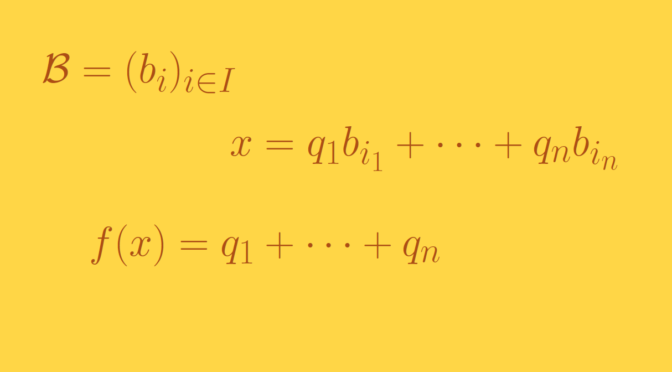A function $$f$$ defined on $$\mathbb R$$ into $$\mathbb R$$ is said to be additive if and only if for all $$x, y \in \mathbb R$$
$f(x+y) = f(x) + f(y).$ If $$f$$ is supposed to be continuous at zero, $$f$$ must have the form $$f(x)=cx$$ where $$c=f(1)$$. This can be shown using following steps:

• $$f(0) = 0$$ as $$f(0) = f(0+0)= f(0)+f(0)$$.
• For $$q \in \mathbb N$$ $$f(1)=f(q \cdot \frac{1}{q})=q f(\frac{1}{q})$$. Hence $$f(\frac{1}{q}) = \frac{f(1)}{q}$$. Then for $$p,q \in \mathbb N$$, $$f(\frac{p}{q}) = p f(\frac{1}{q})= f(1) \frac{p}{q}$$.
• As $$f(-x) = -f(x)$$ for all $$x \in\mathbb R$$, we get that for all rational number $$\frac{p}{q} \in \mathbb Q$$, $$f(\frac{p}{q})=f(1)\frac{p}{q}$$.
• The equality $$f(x+y) = f(x) + f(y)$$ implies that $$f$$ is continuous on $$\mathbb R$$ if it is continuous at $$0$$.
• We can finally conclude to $$f(x)=cx$$ for all real $$x \in \mathbb R$$ as the rational numbers are dense in $$\mathbb R$$.

We’ll use a Hamel basis to construct a discontinuous linear function. The set $$\mathbb R$$ can be endowed with a vector space structure over $$\mathbb Q$$ using the standard addition and the multiplication by a rational for the scalar multiplication.

Using the axiom of choice, one can find a (Hamel) basis $$\mathcal B = (b_i)_{i \in I}$$ of $$\mathbb R$$ over $$\mathbb Q$$. That means that every real number $$x$$ is a unique linear combination of elements of $$\mathcal B$$: $x= q_1 b_{i_1} + \dots + q_n b_{i_n}$ with rational coefficients $$q_1, \dots, q_n$$. The function $$f$$ is then defined as $f(x) = q_1 + \dots + q_n.$ The linearity of $$f$$ follows from its definition. $$f$$ is not continuous as it only takes rational values which are not all equal. And one knows that the image of $$\mathbb R$$ under a continuous map is an interval.